# Plotting Earth relief

Plotting a map of Earth relief can use the data accessed by the `pygmt.datasets.load_earth_relief` function. The data can then be plotted using the `pygmt.Figure.grdimage` method.

```import pygmt
```

Load sample Earth relief data for the entire globe at a resolution of 1 arc-degree. The other available resolutions are shown at https://www.generic-mapping-tools.org/remote-datasets/earth-relief.html.

```grid = pygmt.datasets.load_earth_relief(resolution="01d")
```

## Create a plot

The `pygmt.Figure.grdimage` method takes the `grid` input to create a figure. It creates and applies a color palette to the figure based upon the z-values of the data. By default, it plots the map with the turbo CPT, an equidistant cylindrical projection, and with no frame.

```fig = pygmt.Figure()
fig.grdimage(grid=grid)
fig.show()
```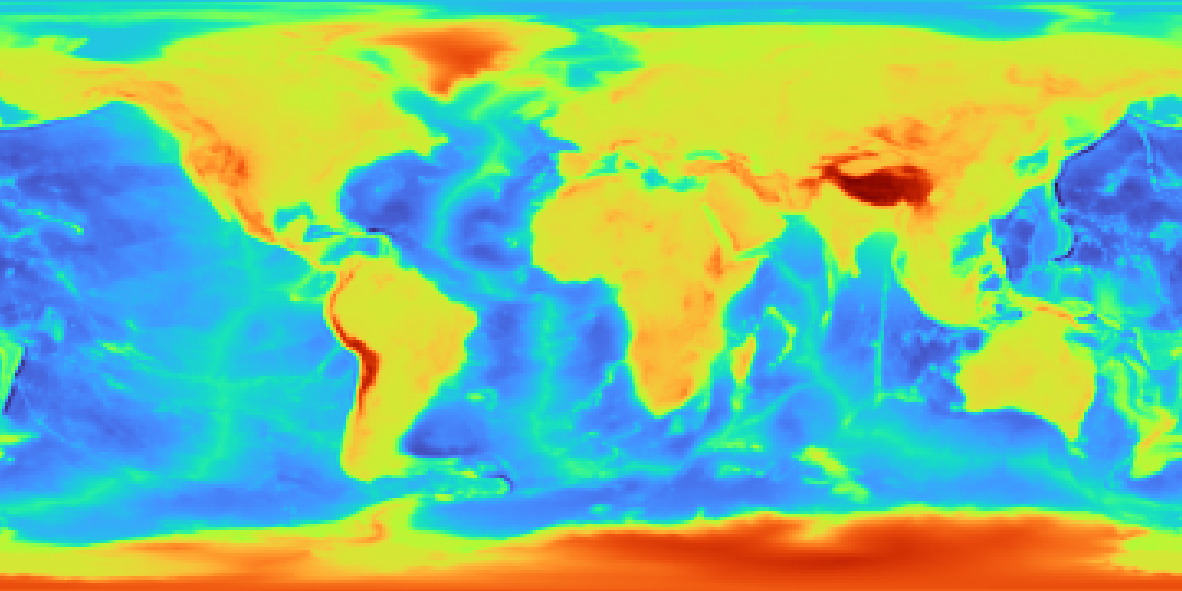`pygmt.Figure.grdimage` can take the optional parameter `projection` for the map. In the example below, `projection` is set to `"R12c"` for a 12-centimeters-wide figure with a Winkel Tripel projection. For a list of available projections, see https://docs.generic-mapping-tools.org/6.4/cookbook/map-projections.html.

```fig = pygmt.Figure()
fig.grdimage(grid=grid, projection="R12c")
fig.show()
```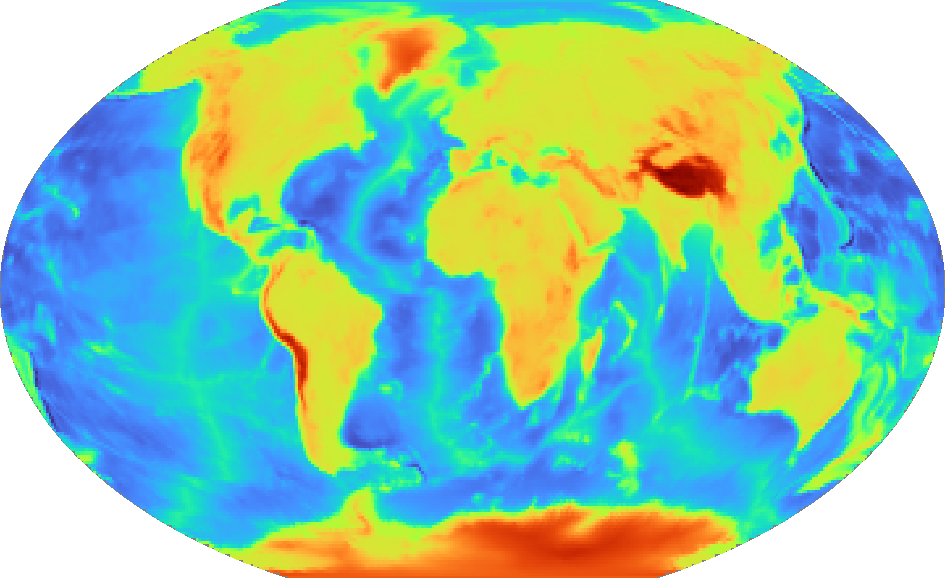## Set a color map

`pygmt.Figure.grdimage` takes the `cmap` parameter to set the CPT of the figure. Examples of common CPTs for Earth relief are shown below. A full list of CPTs can be found at https://docs.generic-mapping-tools.org/6.4/cookbook/cpts.html.

Using the geo CPT:

```fig = pygmt.Figure()
fig.grdimage(grid=grid, projection="R12c", cmap="geo")
fig.show()
```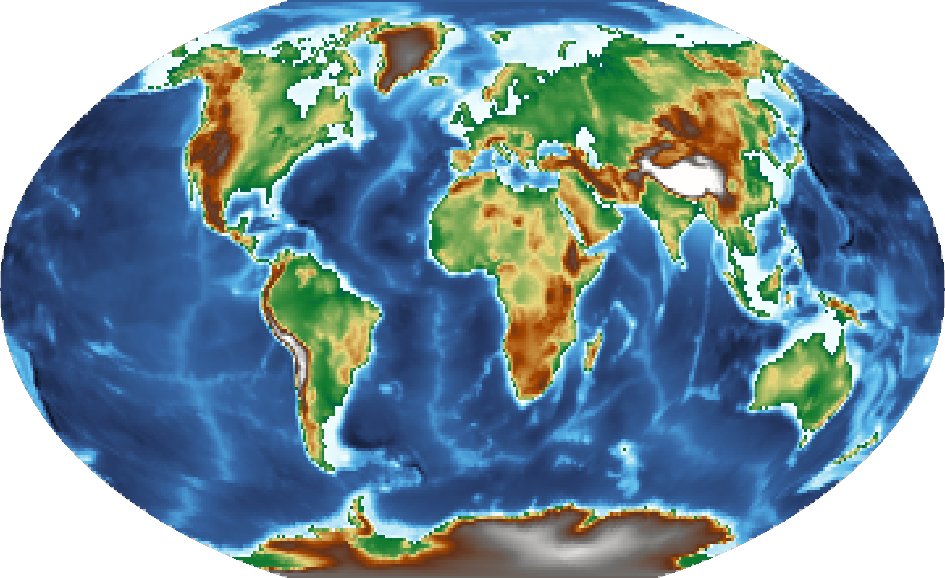Using the relief CPT:

```fig = pygmt.Figure()
fig.grdimage(grid=grid, projection="R12c", cmap="relief")
fig.show()
```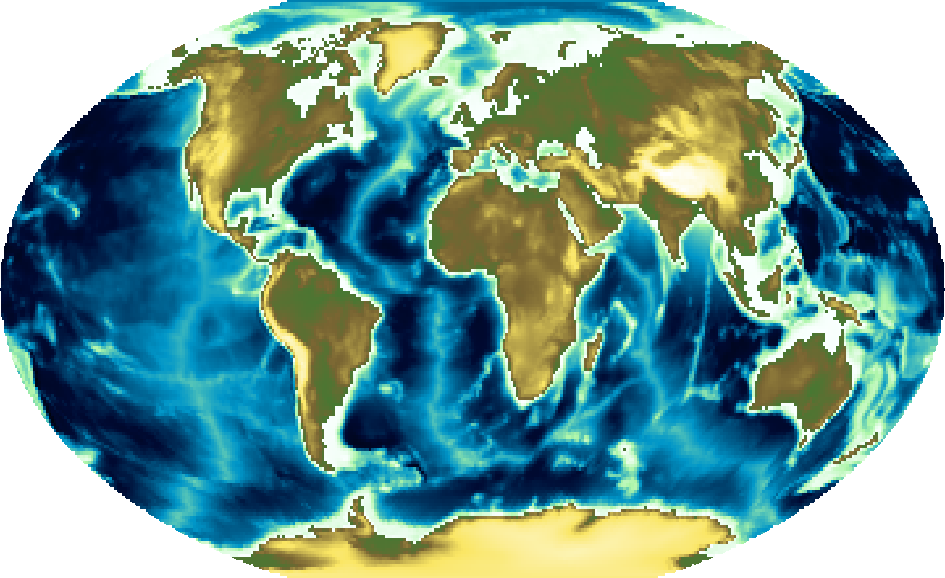The `pygmt.Figure.colorbar` method displays the CPT and the associated Z-values of the figure, and by default uses the same CPT set by the `cmap` parameter for `pygmt.Figure.grdimage`. The `frame` parameter for `pygmt.Figure.colorbar` can be used to set the axis intervals and labels. A list is used to pass multiple arguments to `frame`. In the example below, `"a2500"` sets the axis interval to 2,500, `"x+lElevation"` sets the x-axis label, and `"y+lm"` sets the y-axis label.

```fig = pygmt.Figure()
fig.grdimage(grid=grid, projection="R12c", cmap="geo")
fig.colorbar(frame=["a2500", "x+lElevation", "y+lm"])
fig.show()
```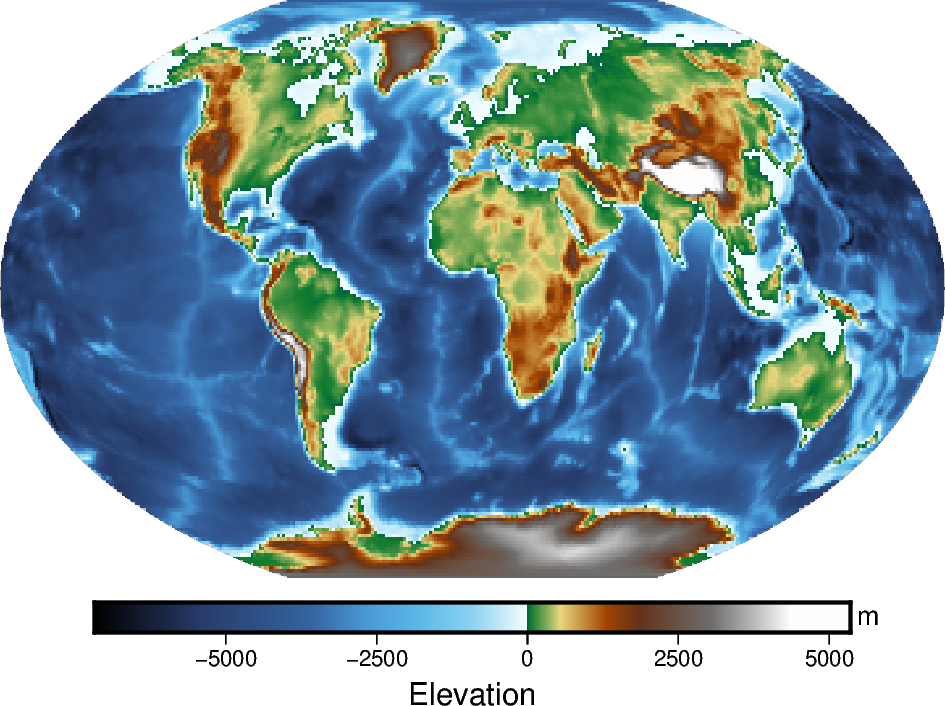## Create a region map

In addition to providing global data, the `region` parameter for `pygmt.datasets.load_earth_relief` can be used to provide data for a specific area. The `region` parameter is required for resolutions at 5 arc-minutes or higher, and accepts a list (as in the example below) or a string. For the later, the geographic range is passed as “xmin/xmax/ymin/ymax”.

The example below uses data with a 10 arc-minutes resolution, and plots it on a 15-centimeters-wide figure with a Mercator projection and a CPT set to geo. `frame="a"` is used to add a frame with annotations to the figure.

```grid = pygmt.datasets.load_earth_relief(resolution="10m", region=[-14, 30, 35, 60])
fig = pygmt.Figure()
fig.grdimage(grid=grid, projection="M15c", frame="a", cmap="geo")
fig.colorbar(frame=["a1000", "x+lElevation", "y+lm"])
fig.show()
```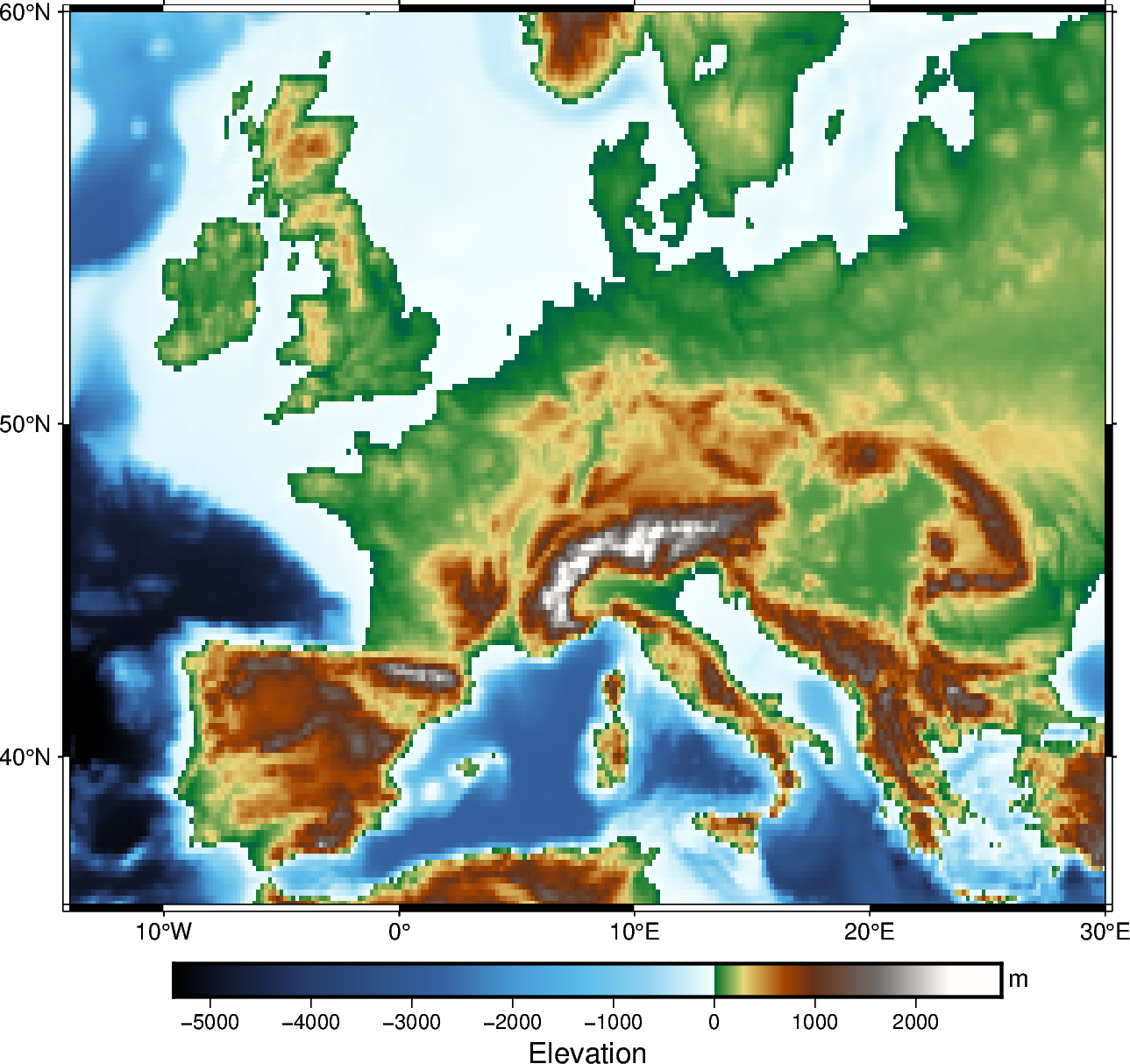Total running time of the script: (0 minutes 4.054 seconds)

Gallery generated by Sphinx-Gallery